# SPSS tutorials

BASICS DATA ANALYSIS T-TEST ANOVA CHI-SQUARE TEST

# SPSS Paired Samples T Test

SPSS paired samples t-test is a procedure for testing whether the means of two metric variables are equal in some population. Both variables have been measured on the same cases. Although “paired samples” suggests that multiple samples are involved, there's really only one sample and two variables. The screenshot below illustrates the basic idea.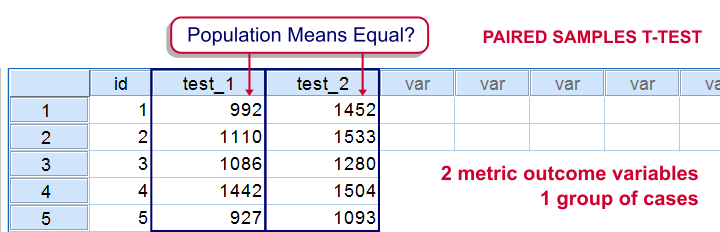## SPSS Paired Samples T-Test Example

A behavioral scientist wants to know whether drinking a single glass of beer affects reaction times. She has 30 participants perform some tasks before and after having a beer and records their reaction times. For each participant she calculates the average reaction time over tasks both before and after the beer, resulting in reaction_times.sav. Can we conclude from these data that a single beer affects reaction time? We'll first open the data by running the syntax below.

*1. Set default directory.

*2. Open data.

get file "reaction_times.sav".

## Quick Data Check

We first just want to know what the data look like. We could do so by taking a look at the histograms of the two variables. However, a nice alternative for two variables measured on the same respondents is a scatter plot. The screenshots below walk you through.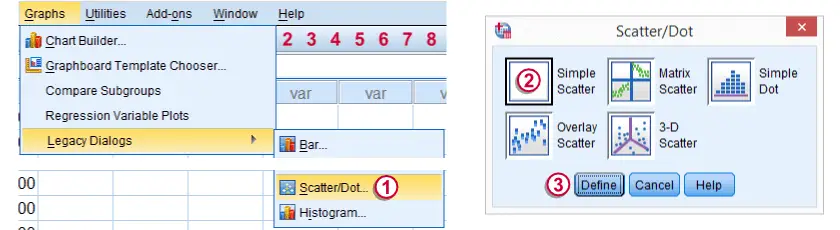We first navigate to GraphsLegacy DialogsScatter/Dot...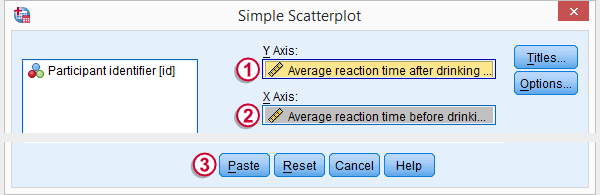We then move `reac_1` and `reac_2` to X-Axis and Y-Axis. Clicking results in the syntax below.

*Run scatter plot.

GRAPH
/SCATTERPLOT(BIVAR)=reac_2 WITH reac_1
/MISSING=LISTWISE.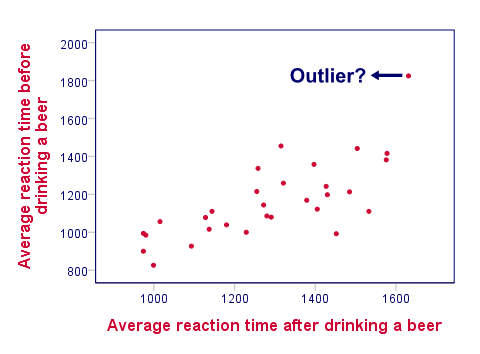Normal reactions times are between 800 and 1500 ms (= milliseconds). Neither variable has any values that are way out of this normal range so the data seem plausible.
We also see a substantial positive correlation between the variables; respondents who were fast on the first task tend to be fast on the second task as well. The graph seems to suggest that the mean reaction time before a beer is somewhere near 1100 ms (vertical axis) and after a beer perhaps 1300 ms (horizontal axis).
One respondent (right top corner, denoted “outlier”) is remarkably slow compared to the others. However, we decide that its scores are not extreme enough to justify removing it from the data.

## 2. Assumptions Paired Samples T-Test

SPSS will happily provide us with test results but we can only take those seriously insofar as the assumptions for our test are met. For the paired samples t-test, these are

• independent observations or, more precisely, independent and identically distributed variables;
• the difference scores between the two variables must be normally distributed in our population.

The first assumption is often satisfied if each case (row of data values) holds a distinct person or other unit of analysis. The normality assumption is mostly relevant for small sample sizes (say N < 30). If it's violated, consider a Wilcoxon signed-ranks test instead of a t-test. However, our data seems to meet both assumptions so we'll proceed to the t-test.

## 3. Run SPSS Paired Samples T-Test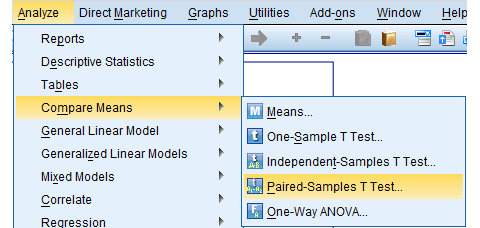We'll first navigate to AnalyzeCompare MeansPaired-Samples T Test.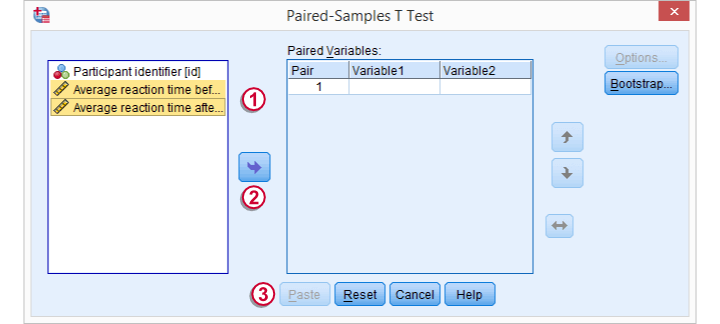Select both variables andmove them into the Paired Variables box.Clicking results in the syntax below.

*Run paired samples t-test.

T-TEST PAIRS=reac_1 WITH reac_2 (PAIRED)
/CRITERIA=CI(.9500)
/MISSING=ANALYSIS.

## 4. SPSS Paired Samples T-Test Output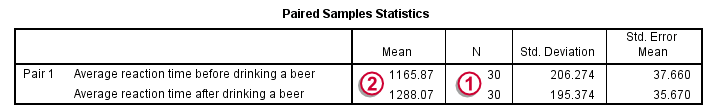The first table (“Paired Samples Statistics”) presents the descriptive statistics we'll report. (Do not use the `DESCRIPTIVES` command for obtaining these.The reason for this is that the significance test is (necessarily) limited to cases without any missing values on the test variables. Cases with a missing value on one of the test variables should therefore be excluded from the descriptives as well. This holds for the descriptives from the paired samples t-test but not for `DESCRIPTIVES`.)Since N = 30, we don't have any missing values on the test variables andas expected, the mean reaction time before a beer (1166 ms) is lower than after a beer (1288 ms).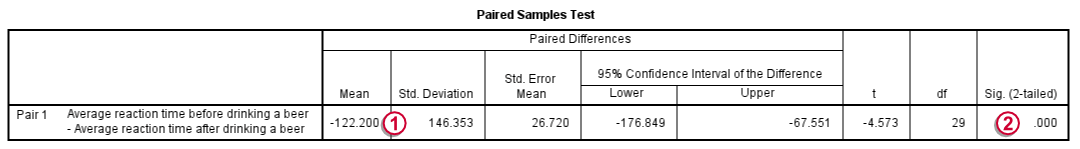On average, respondents slow down some 122 ms. We could have calculated this from the first table ourselves.The p-value denoted by “Sig. (2-tailed)” is 0. (If we double-click it, we'll see it's precisely 0.000083, meaning a .0083 % chance.) So if the population means are equal, there's a 0% chance of finding this result. We therefore reject the null hypothesis. Even a single beer slows people down on the given tasks.
Note that the p-value is two-sided. This means that the p-value consists of a .00415% chance of finding a difference < -122 ms and another .00415% chance of finding a difference > 122 ms.

## 5.Reporting a Paired Samples T-Test

As we mentioned before, we'll always report the descriptive statistics obtained from the paired samples t-test. For the significance test, we may write something like “Participants became slower after drinking a single beer, t(29) = -4.6, p = 0.00”.

# Let me know what you think!

*Required field. Your comment will show up after approval from a moderator.

# This tutorial has 15 comments

•### By Naod on January 9th, 2020

excellent

•### By Naod on January 9th, 2020

very good explanation

•### By Naod on January 9th, 2020

well explained!

•### By Teshale Tefera on February 19th, 2019

It is good way of instructing and it is better if you test your customers knowledge and skills on different areas of higher statistics supplemented with certificate. I expect.

•### By dr muhamad ali rahni on September 2nd, 2018

I'm not into statistics but I do supervise MS students. Your interpretation of SPSS output on repeated measures is by far the best. Thanx from K.L. Msia!